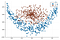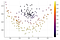# Deep Learning with Julia

## A brief tutorial on training a Neural Network with Flux.jl

Flux.jl is the most popular Deep Learning framework in Julia. It provides a very elegant way of programming Neural Networks. Unfortunately, since Julia is still not as popular as Python, there aren’t as many tutorial guides on how to use it. Also, Julia is improving very fast, so things can change a lot in a short amount of time.

I’ve been trying to learn Flux.jl for a while, and I realized that most tutorials out there are actually outdated. So this is a brief updated tutorial.

# 1. What we are going to build

So, the goal of this tutorial is to build a simple classification Neural Network. This will be enough for anyone who is interested in using Flux. After learning the very basics, the rest is pretty much altering Networks architectures and loss functions.

# 2. Generating our Dataset

Instead of importing data from somewhere, let’s do everything self-contained. Hence, we write two auxiliary functions to generate our data:

`#Auxiliary functions for generating our datafunction generate_real_data(n)    x1 = rand(1,n) .- 0.5    x2 = (x1 .* x1)*3 .+ randn(1,n)*0.1    return vcat(x1,x2)endfunction generate_fake_data(n)    θ  = 2*π*rand(1,n)    r  = rand(1,n)/3    x1 = @. r*cos(θ)    x2 = @. r*sin(θ)+0.5    return vcat(x1,x2)end# Creating our datatrain_size = 5000real = generate_real_data(train_size)fake = generate_fake_data(train_size)# Visualizingscatter(real[1,1:500],real[2,1:500])scatter!(fake[1,1:500],fake[2,1:500])`Visualizing the Dataset

# 3. Creating the Neural Network

The creation of Neural Network architectures with Flux.jl is very direct and clean (cleaner than any other Library I know). Here is how you do it:

`function NeuralNetwork()    return Chain(            Dense(2, 25,relu),            Dense(25,1,x->σ.(x))            )end`

The code is very self-explanatory. The first layer is a dense layer with input 2, output 25 and relu for activation function. The second is a dense layer with input 25, output 1 and a sigmoid activation function. The `Chain` ties the layers together. Yeah, it’s that simple.

# 4. Training our Model

Next, let’s prepare our model to be trained.

`# Organizing the data in batchesX    = hcat(real,fake)Y    = vcat(ones(train_size),zeros(train_size))data = Flux.Data.DataLoader(X, Y', batchsize=100,shuffle=true);# Defining our model, optimization algorithm and loss functionm    = NeuralNetwork()opt = Descent(0.05)loss(x, y) = sum(Flux.Losses.binarycrossentropy(m(x), y))`

In the code above, we first organize our data into one single dataset. We use the `DataLoader` function from Flux, that helps us create the batches and shuffles our data. Then, we call our model and define the loss function and the optimization algorithm. In this example, we are using gradient descent for optimization and cross-entropy for the loss function.

Everything is ready, and we can start training the model. Here, I’ll show two way of doing it.

## Training Method 1

`ps = Flux.params(m)epochs = 20for i in 1:epochs    Flux.train!(loss, ps, data, opt)endprintln(mean(m(real)),mean(m(fake))) # Print model prediction`

In this code, first we declare what parameters are going to be trained, which is done using the `Flux.params()` function. The reason for this is that we can choose not to train a layer in our network, which might be useful in the case of transfer learning. Since in our example we are training the whole model, we just pass all the parameters to the training function.

Other then this, there is not much to be said. The final line of code is just printing the mean prediction probability our model is giving.

## Training Method 2

`m    = NeuralNetwork()function trainModel!(m,data;epochs=20)    for epoch = 1:epochs        for d in data            gs = gradient(Flux.params(m)) do                l = loss(d...)            end            Flux.update!(opt, Flux.params(m), gs)        end    end    @show mean(m(real)),mean(m(fake))endtrainModel!(m,data;epochs=20)`

This method is a bit more convoluted, because we are doing the training “manually”, instead of using the training function given by Flux. This is interesting since one has more control over the training, which can be useful for more personalized training methods. Perhaps the most confusing part of the code is this one:

`gs = gradient(Flux.params(m)) do     l = loss(d...)endFlux.update!(opt, Flux.params(m), gs)`

The function `gradient` receives the parameters to which it will calculate the gradient, and applies it to the loss function, that is calculated for the batch `d`. The splater operator (the three dots) is just a neat way of passing x and y to the loss function. Finally, the `update!` function is adjusting the parameters according to the gradients, which are stored in the variable `gs`.

# 5. Visualizing the Results

Finally, the model is trained, and we can visualize it’s performance again the dataset.

`scatter(real[1,1:100],real[2,1:100],zcolor=m(real)')scatter!(fake[1,1:100],fake[2,1:100],zcolor=m(fake)',legend=false)`Neural Network prediction again the training dataset

Note that our model is performing quite well, it can properly classify the points in the middle with probability close to 0, implying that it belongs to the “fake data”, while the rest has probability close to 1, meaning that it belongs to the “real data”.

# 6. Conclusion

That’s all for our brief introduction. Hopefully this is a first article on a series on how to do Machine Learning with Julia.

Note that this tutorial is focused on simplicity, and not on writing the most efficient code. For that learning how to improve performance, look here.

TL;DR
Here is the code with everything put together:

`#Auxiliary functions for generating our datafunction generate_real_data(n)    x1 = rand(1,n) .- 0.5    x2 = (x1 .* x1)*3 .+ randn(1,n)*0.1    return vcat(x1,x2)endfunction generate_fake_data(n)    θ  = 2*π*rand(1,n)    r  = rand(1,n)/3    x1 = @. r*cos(θ)    x2 = @. r*sin(θ)+0.5    return vcat(x1,x2)end# Creating our datatrain_size = 5000real = generate_real_data(train_size)fake = generate_fake_data(train_size)# Visualizingscatter(real[1,1:500],real[2,1:500])scatter!(fake[1,1:500],fake[2,1:500])function NeuralNetwork()    return Chain(            Dense(2, 25,relu),            Dense(25,1,x->σ.(x))            )end# Organizing the data in batchesX    = hcat(real,fake)Y    = vcat(ones(train_size),zeros(train_size))data = Flux.Data.DataLoader(X, Y', batchsize=100,shuffle=true);# Defining our model, optimization algorithm and loss functionm    = NeuralNetwork()opt = Descent(0.05)loss(x, y) = sum(Flux.Losses.binarycrossentropy(m(x), y))# Training Method 1ps = Flux.params(m)epochs = 20for i in 1:epochs    Flux.train!(loss, ps, data, opt)endprintln(mean(m(real)),mean(m(fake))) # Print model prediction# Visualizing the model predictionsscatter(real[1,1:100],real[2,1:100],zcolor=m(real)')scatter!(fake[1,1:100],fake[2,1:100],zcolor=m(fake)',legend=false)`

## Coffee in a Klein Bottle

Essays on Mathematics, Programming and everything in between

## Coffee in a Klein Bottle

Coffee in a Klein Bottle is a publication focused on sharing essays/tutorials/notes on Mathematics, Programing and everything in between. The contributors are mainly students or alumni at EMAp/FGV.

Written by

## DSB

Interested in Mathematics, Julia Programming and Mathematica.## Coffee in a Klein Bottle

Coffee in a Klein Bottle is a publication focused on sharing essays/tutorials/notes on Mathematics, Programing and everything in between. The contributors are mainly students or alumni at EMAp/FGV.

## More From Medium

Medium is an open platform where 170 million readers come to find insightful and dynamic thinking. Here, expert and undiscovered voices alike dive into the heart of any topic and bring new ideas to the surface. Learn more

Follow the writers, publications, and topics that matter to you, and you’ll see them on your homepage and in your inbox. Explore

If you have a story to tell, knowledge to share, or a perspective to offer — welcome home. It’s easy and free to post your thinking on any topic. Write on Medium